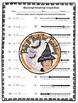# INEQUALITIES Worksheets BUNDLE Add Subtract Multiply Divide Graph Solve MatchSubject
Resource Type
File Type

Zip

(2 MB|8 pages)
Product Rating
Standards
4 Products in this Bundle
4 products
1. Teachers ~ Do you want a GREAT practice for students to write, solve, and graph an inequality? Then this Writing, Solving, Graphing 2-page Worksheet is perfect for you! You'll enjoy using this as guided or independent work, or at a station, year after year!Be sure to L@@K at my other, 675+ TERRIFIC
2. You'll LOVE using this 10 question Matching Graphing Inequalities Worksheet with Answer KEY where students have to match the written Inequality with the graphed Inequality. Great for practicing learning how to graph, read, and write Inequalities. ANSWER KEY INCLUDED. I have LOTS of great INEQUALITIE
3. GREAT practice Adding and Subtracting One-Step Inequalities Solving and Graphing Worksheet with Answer KEY. This is a 10 question practice for solving and graphing add and subtract 1-step inequalities. You'll enjoy using this year after year. Hand made Answer KEY included. Be sure to see my other In
4. Multiply and Divide One-Step INEQUALITIES Solving and Graphing Worksheet with Answer KEY is GREAT practice for students on how to multiply, divide, solve and graph a 1-step inequality. Hand written ANSWER KEY included. You'll love using this year after year to assess this skill. I have this same typ
• Bundle Description
• StandardsNEW

Teachers ~ do you need GREAT worksheets to practice Inequalities? And, would you like to save some money \$? This is a terrific BUNDLE with everything your students need to practice writing, solving, graphing, matching, adding, subtracting, multiplying, and dividing inequalites. You'll enjoy using these during your Inequalities Unit year after year!

Be sure to L@@K at my other 675+ TERRIFIC teaching resources!!!

~ ~ THANK YOU KINDLY ~ ~

Solve word problems leading to inequalities of the form 𝘱𝘹 + 𝘲 > 𝘳 or 𝘱𝘹 + 𝘲 < 𝘳, where 𝘱, 𝘲, and 𝘳 are specific rational numbers. Graph the solution set of the inequality and interpret it in the context of the problem. For example: As a salesperson, you are paid \$50 per week plus \$3 per sale. This week you want your pay to be at least \$100. Write an inequality for the number of sales you need to make, and describe the solutions.
Use variables to represent quantities in a real-world or mathematical problem, and construct simple equations and inequalities to solve problems by reasoning about the quantities.
Write an inequality of the form 𝘹 > 𝘤 or 𝘹 < 𝘤 to represent a constraint or condition in a real-world or mathematical problem. Recognize that inequalities of the form 𝘹 > 𝘤 or 𝘹 < 𝘤 have infinitely many solutions; represent solutions of such inequalities on number line diagrams.
Use variables to represent numbers and write expressions when solving a real-world or mathematical problem; understand that a variable can represent an unknown number, or, depending on the purpose at hand, any number in a specified set.
Understand solving an equation or inequality as a process of answering a question: which values from a specified set, if any, make the equation or inequality true? Use substitution to determine whether a given number in a specified set makes an equation or inequality true.
Total Pages
8 pages
Included
Teaching Duration
N/A
Report this Resource to TpT
Reported resources will be reviewed by our team. Report this resource to let us know if this resource violates TpT’s content guidelines.